# RD Sharma Solutions For Class 12 Maths Exercise 4.14 Chapter 4 Inverse Trigonometric Functions

Our expert team has designed the solutions for RD Sharma Solutions for Class 12 Maths Exercise 4.14 Chapter 4 Inverse Trigonometric Functions to help students prepare for their exams with ease. RD Sharma Solutions for Class 12 is one of the best reference materials for CBSE students. Learners can download the pdf from the links provided below.

Experts suggest students practice the solutions many number of times to yield good results in their exams. In Exercise 4.14 of Chapter 4 Inverse Trigonometric Functions, we shall discuss the ASA congruence condition for two congruent triangles and their properties.

## Download the PDF of RD Sharma Solutions For Class 12 Chapter 4 – Inverse Trigonometric Functions Exercise 4.14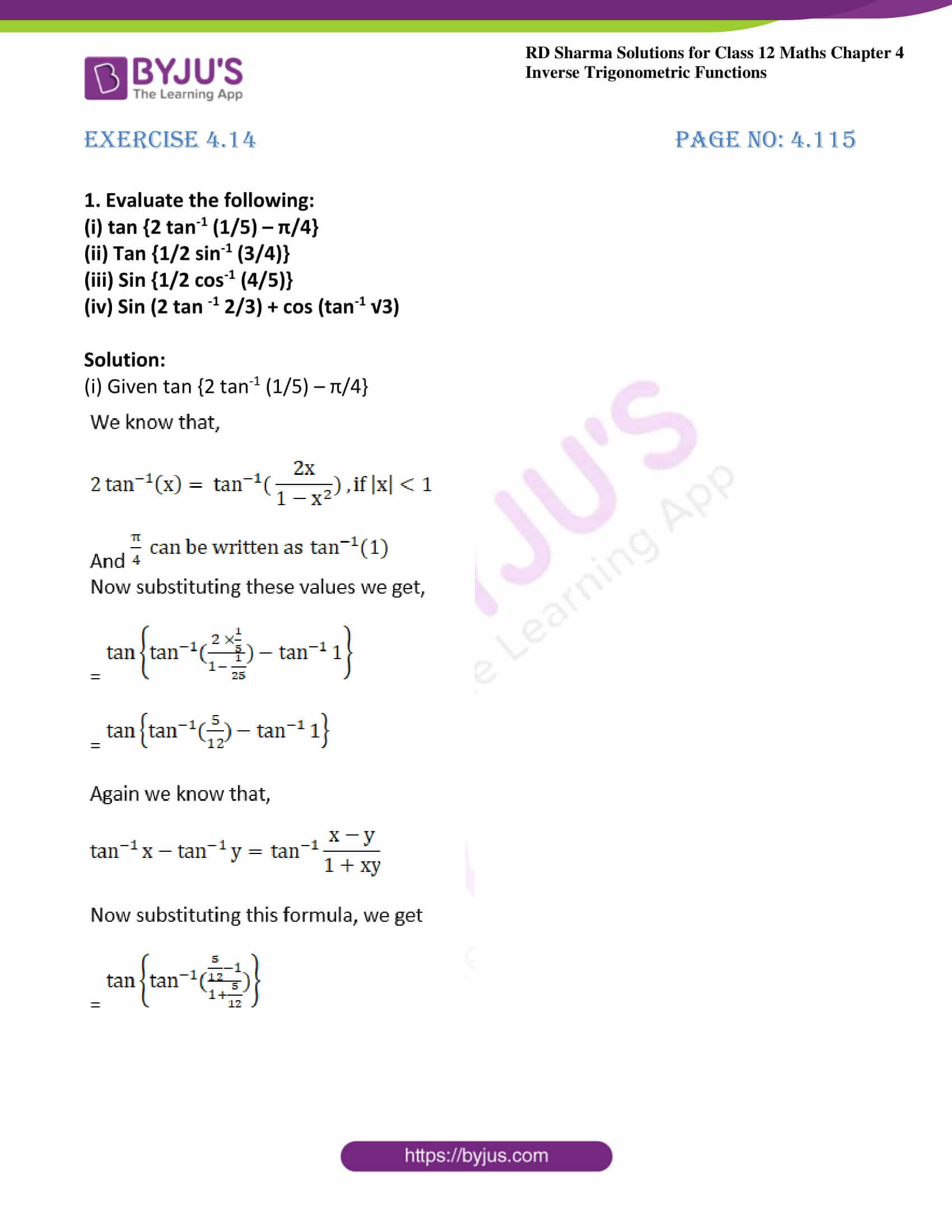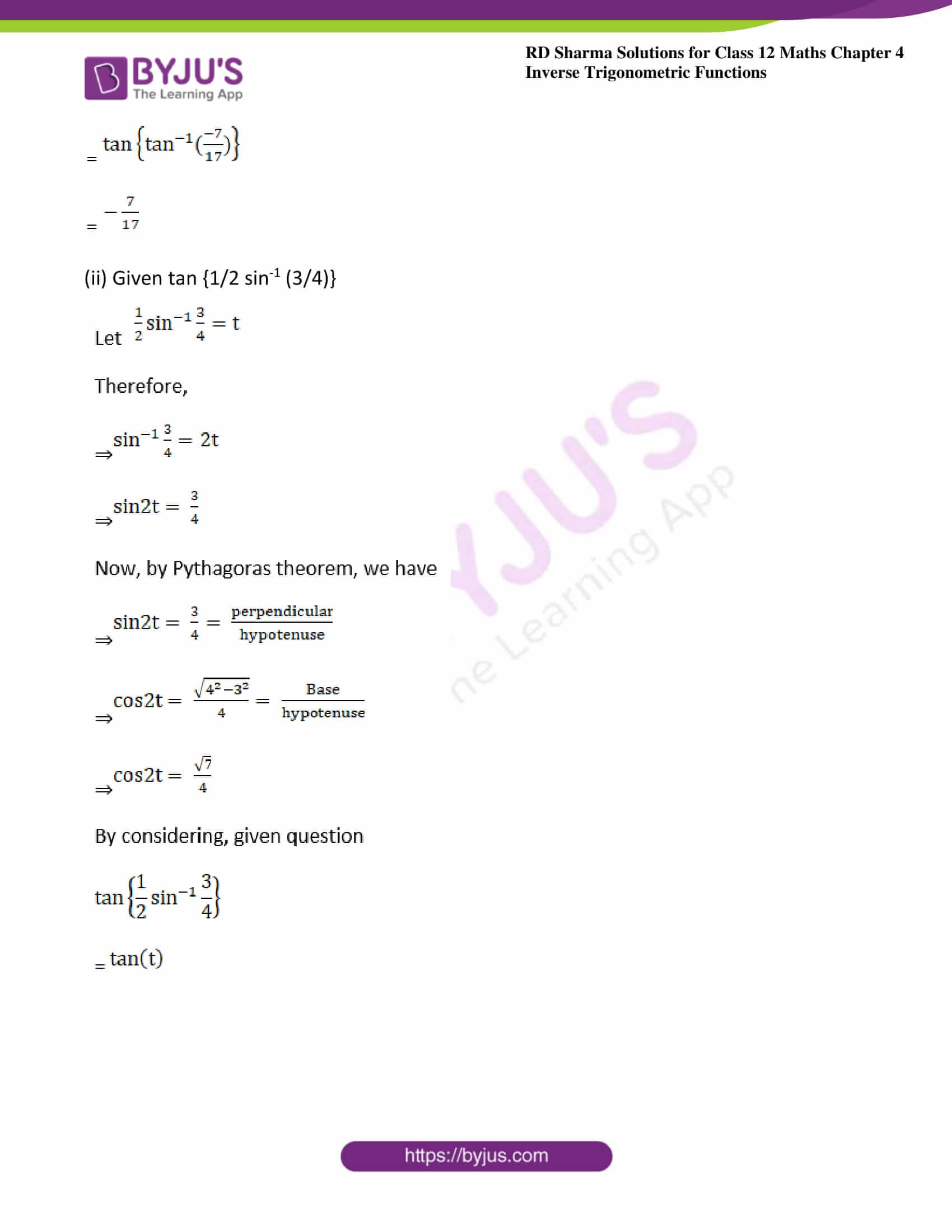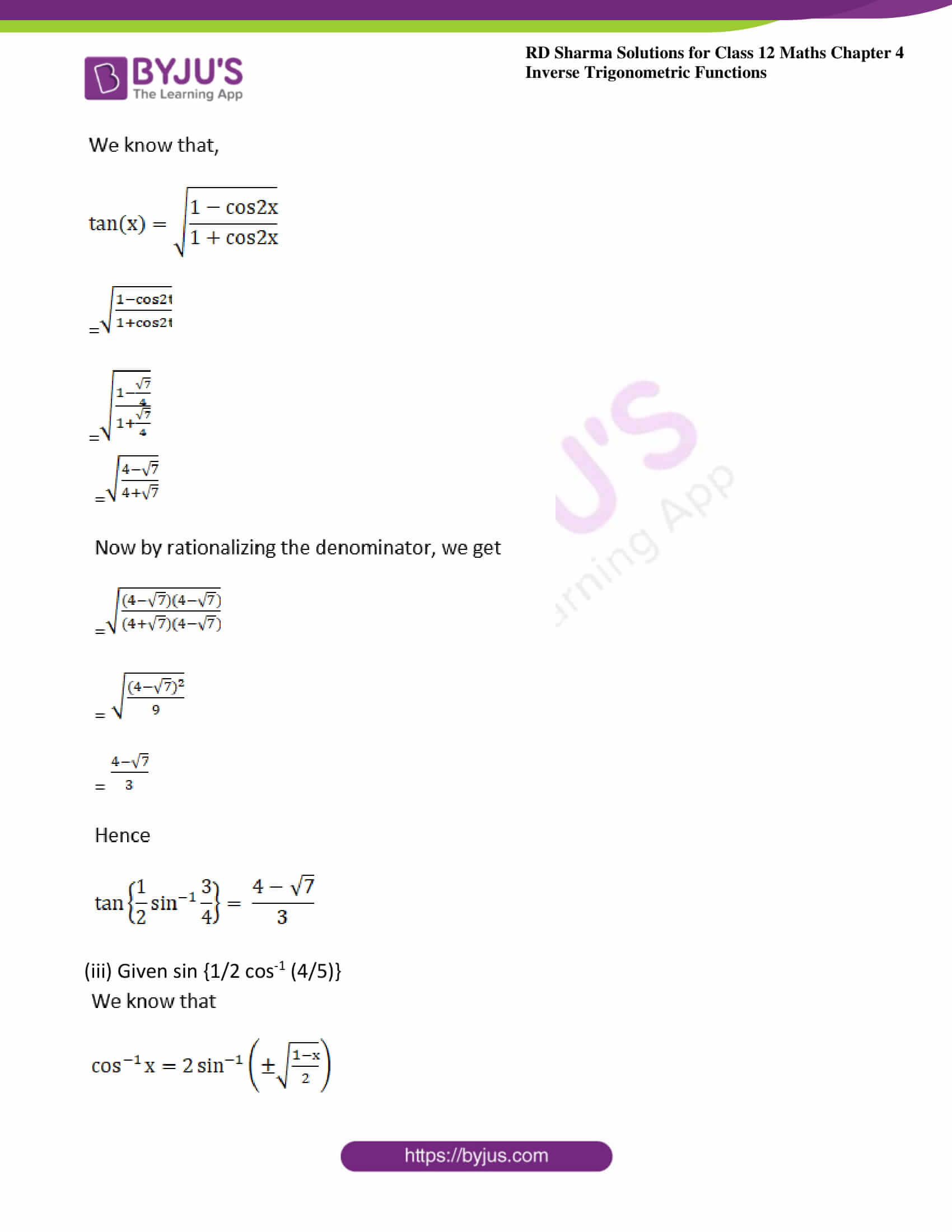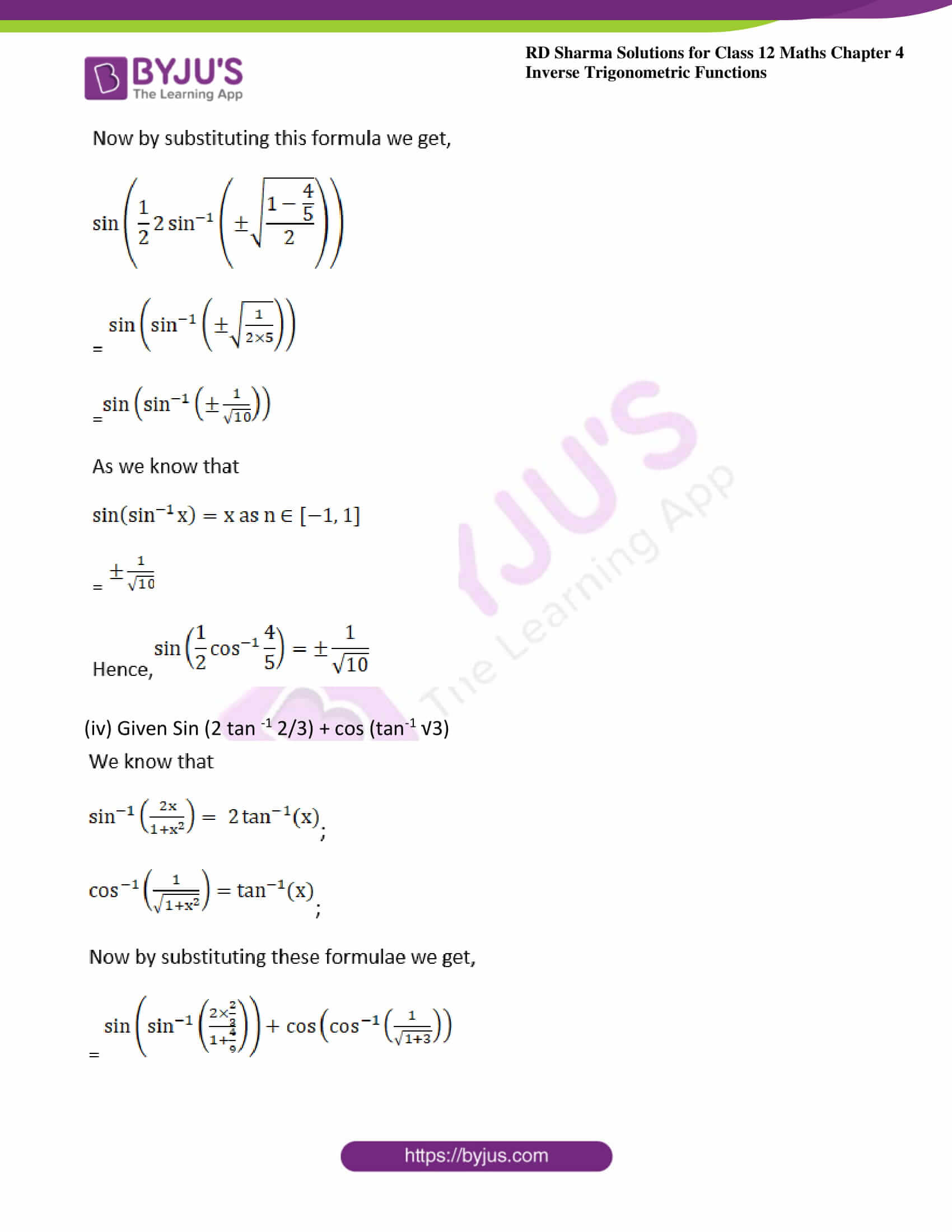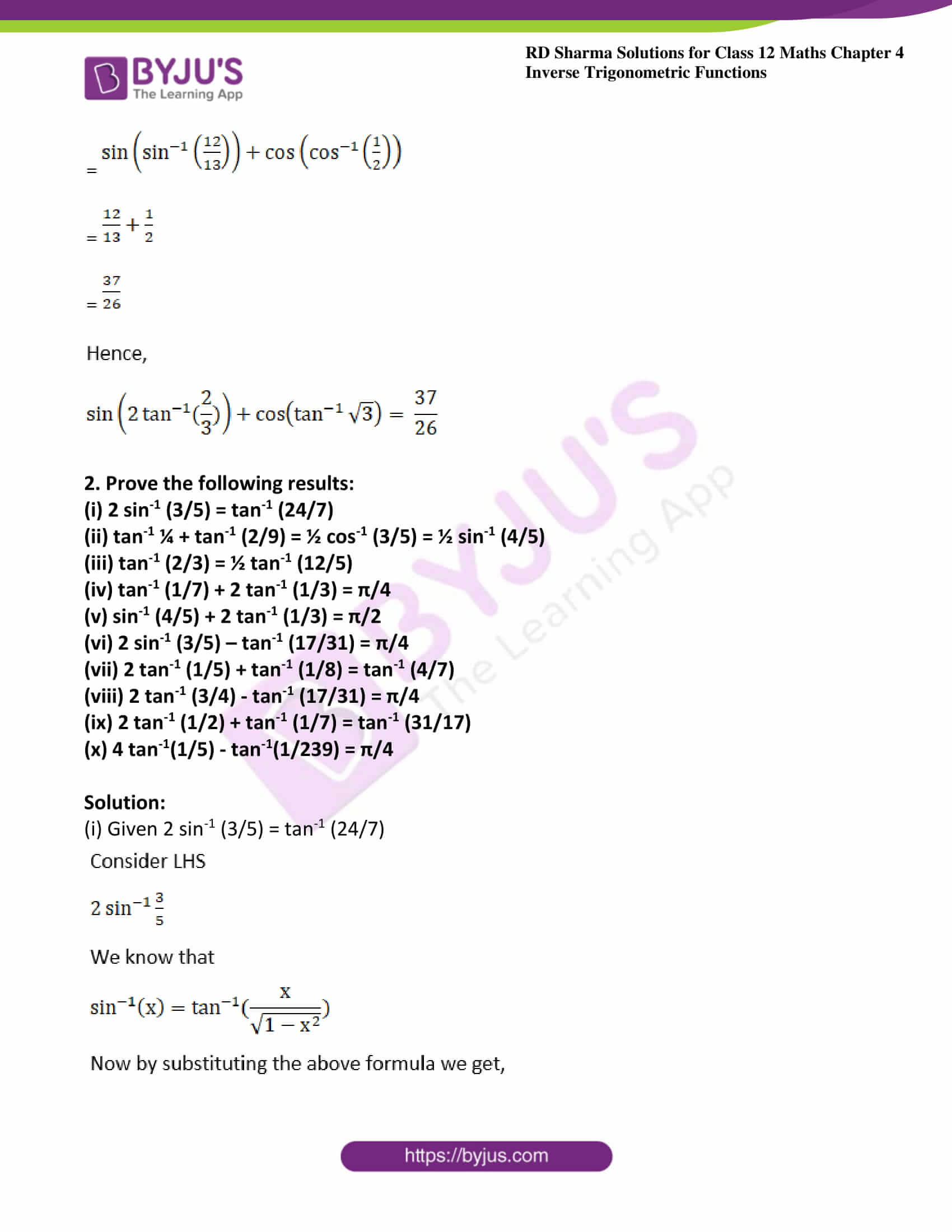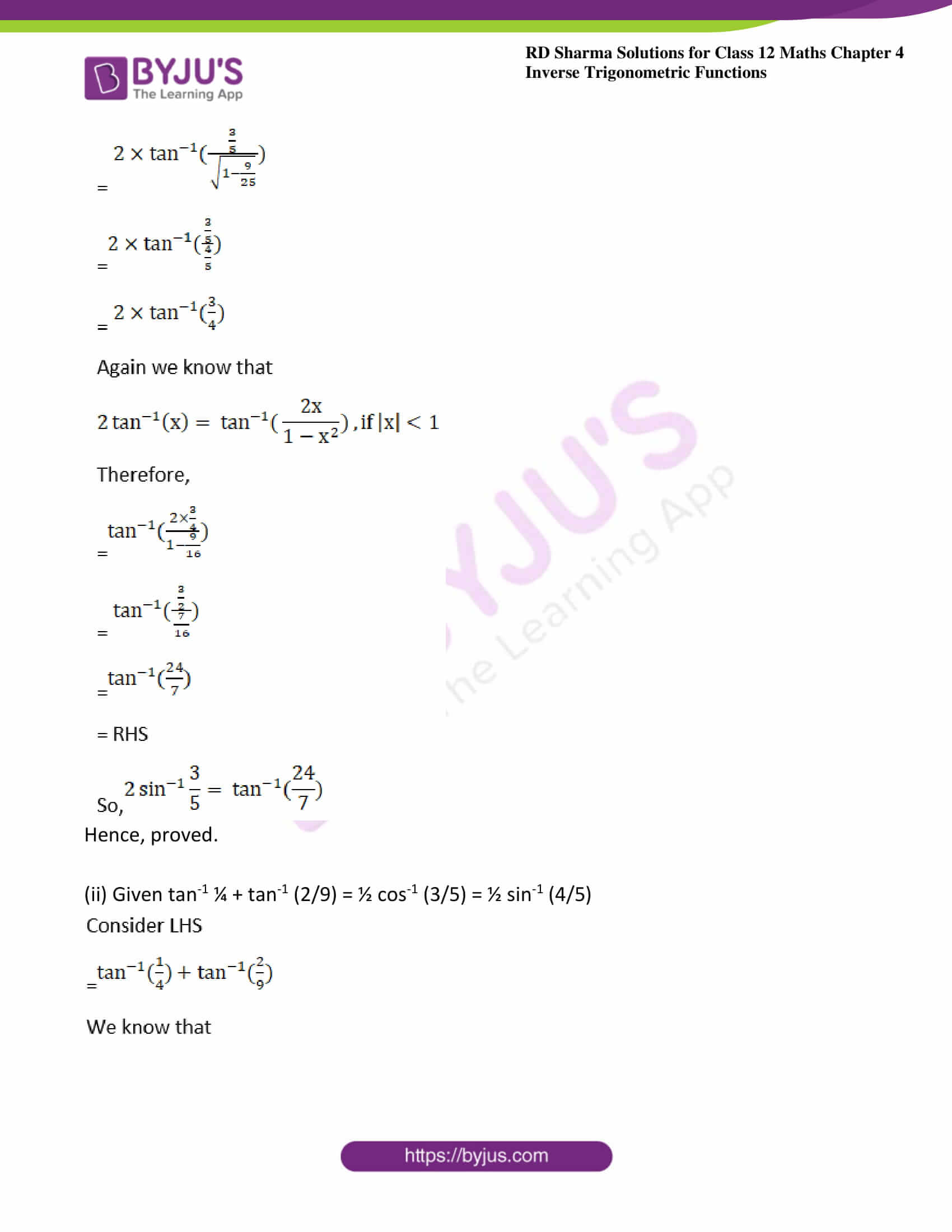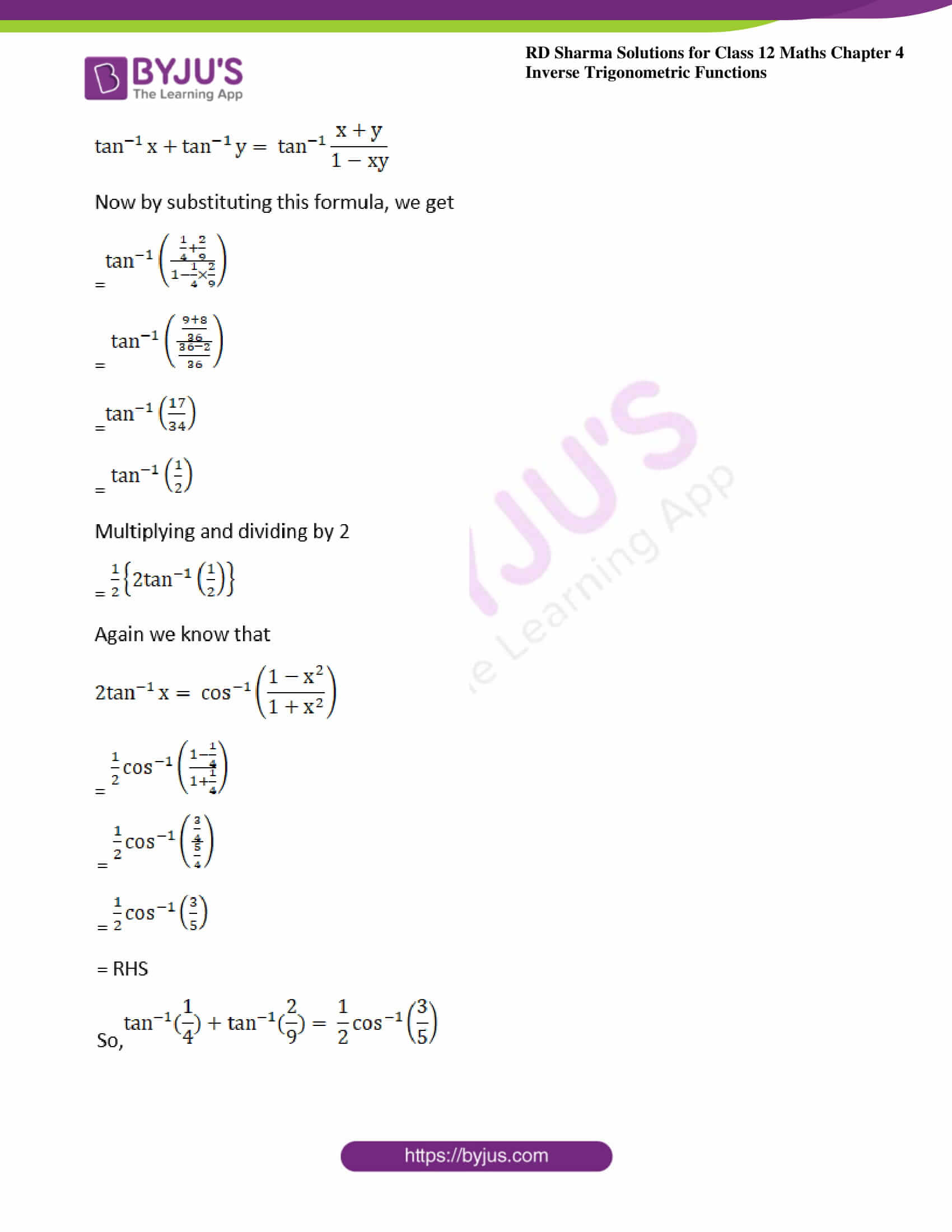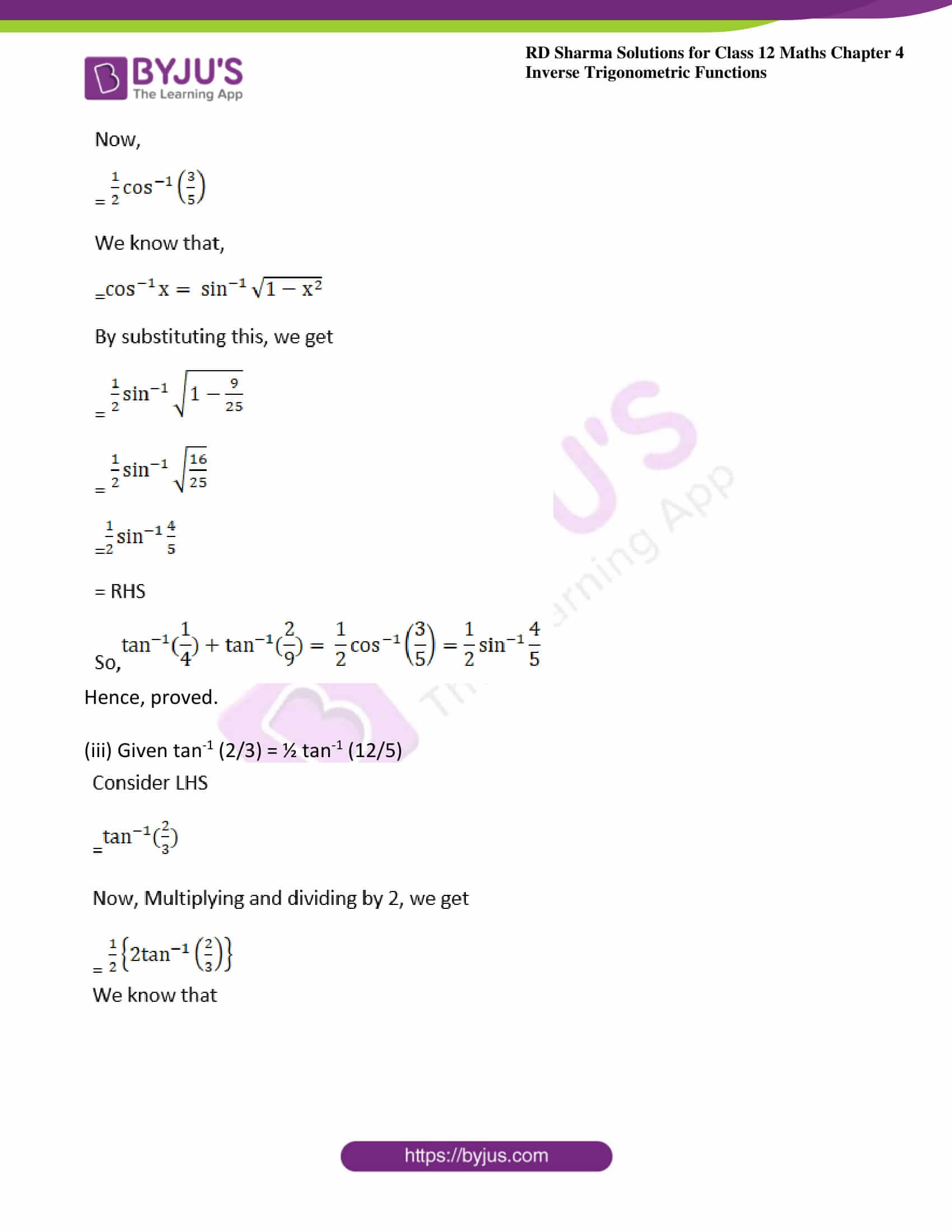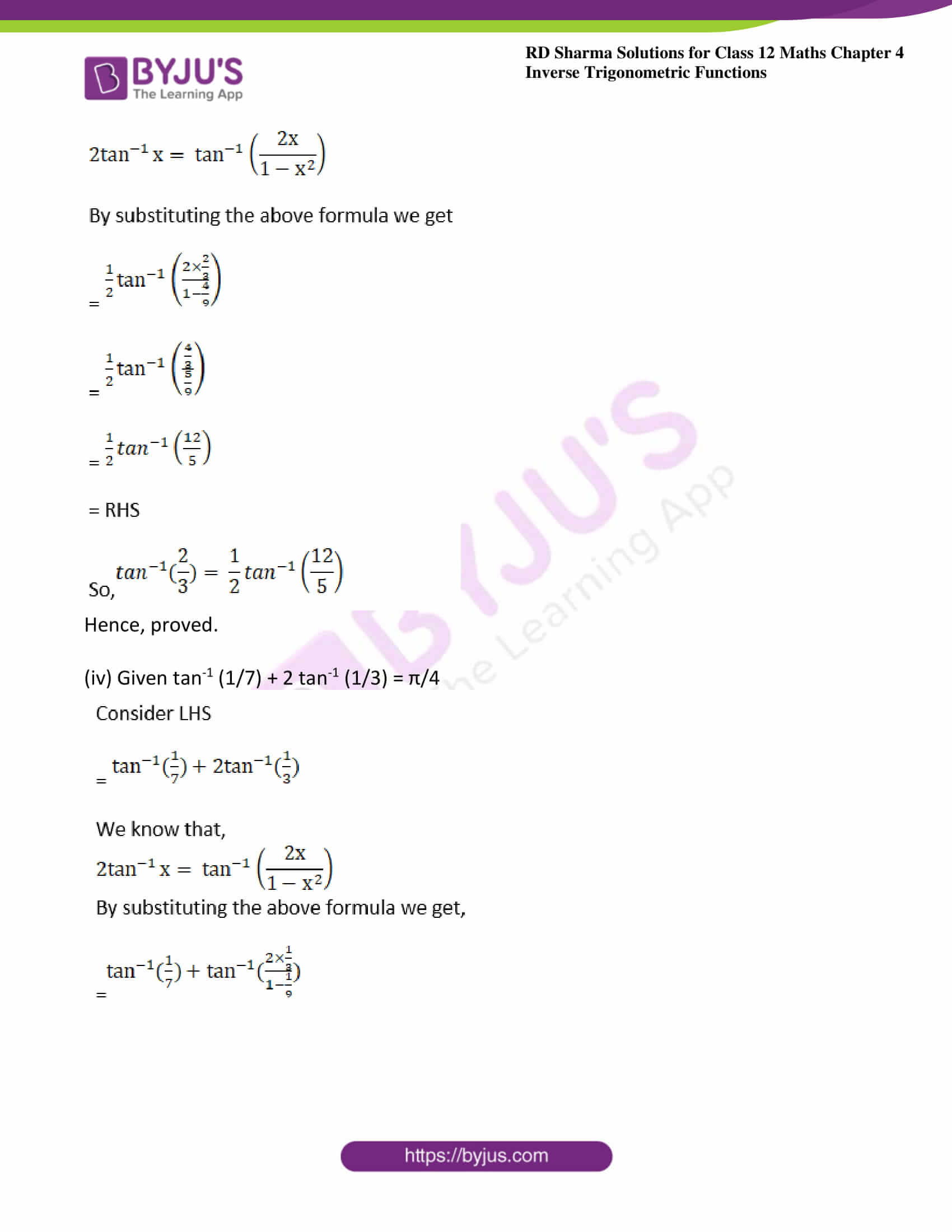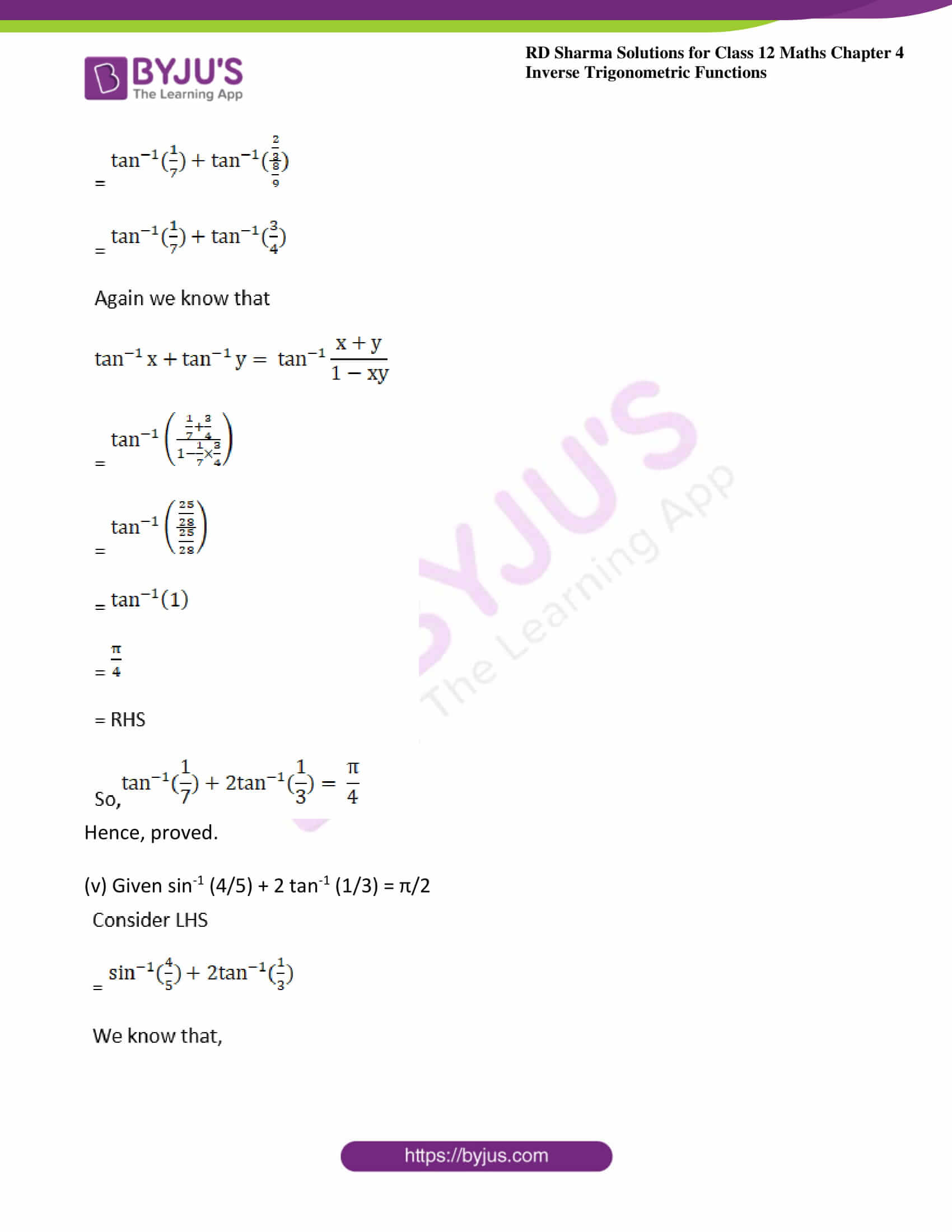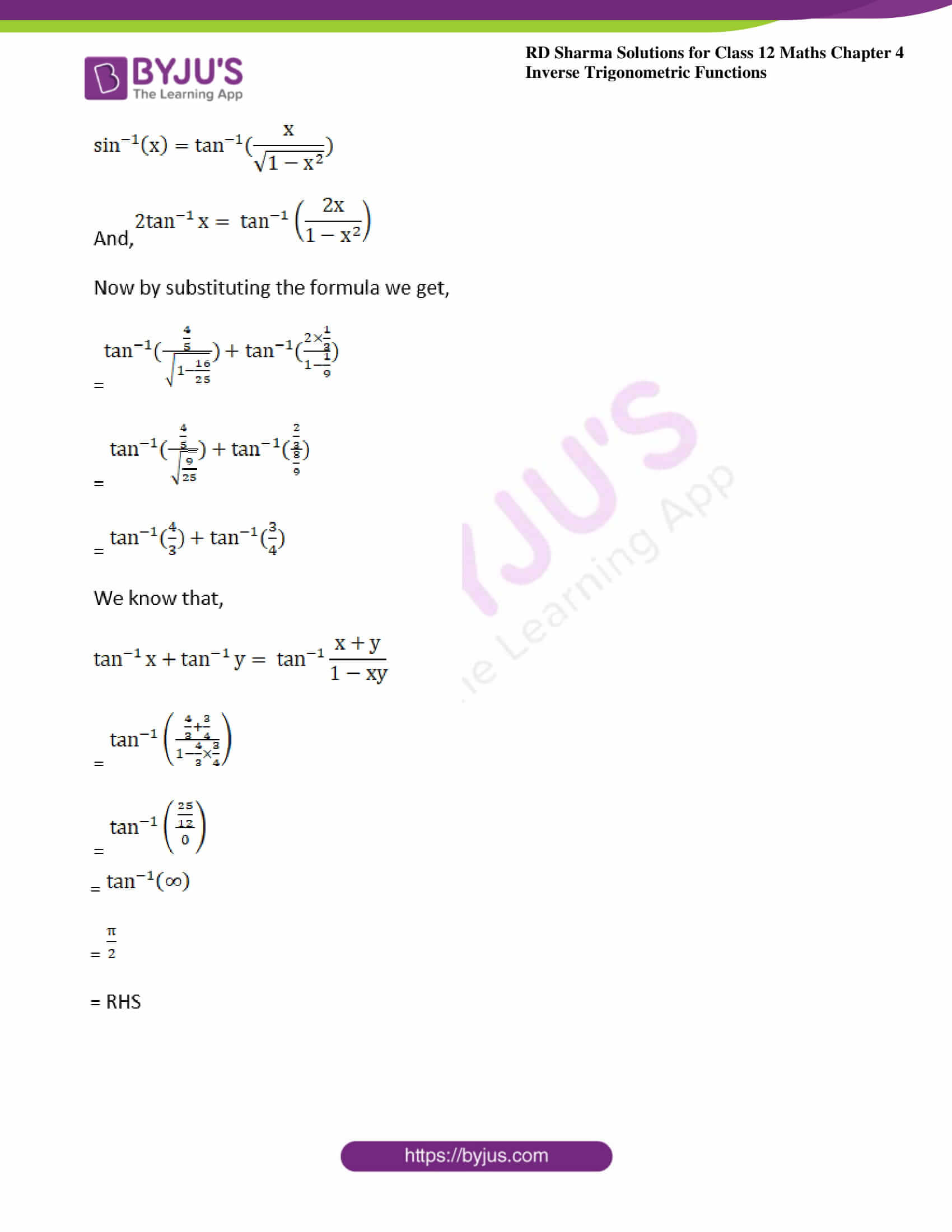### Exercise 4.14 Page No: 4.115

1. Evaluate the following:

(i) tan {2 tan-1 (1/5) – π/4}

(ii) Tan {1/2 sin-1 (3/4)}

(iii) Sin {1/2 cos-1 (4/5)}

(iv) Sin (2 tan -1 2/3) + cos (tan-1 √3)

Solution:

(i) Given tan {2 tan-1 (1/5) – π/4}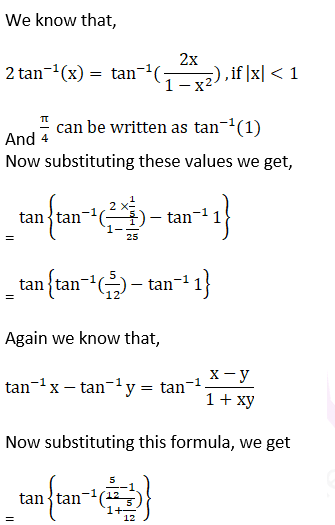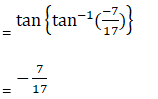(ii) Given tan {1/2 sin-1 (3/4)}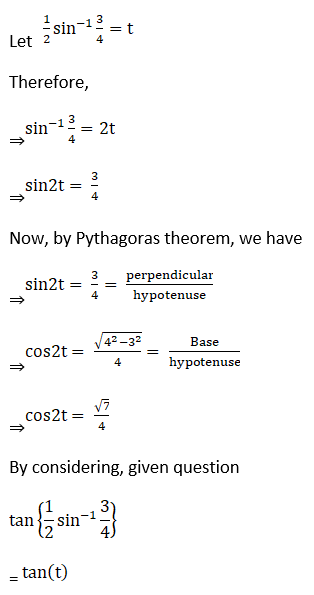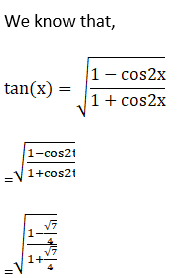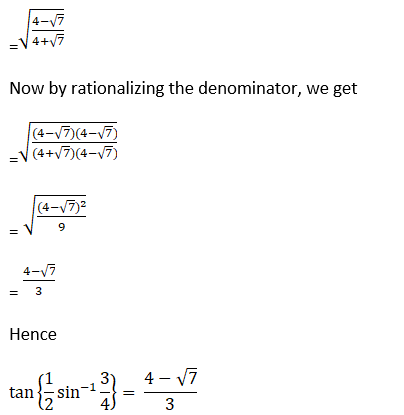(iii) Given sin {1/2 cos-1 (4/5)}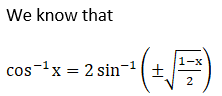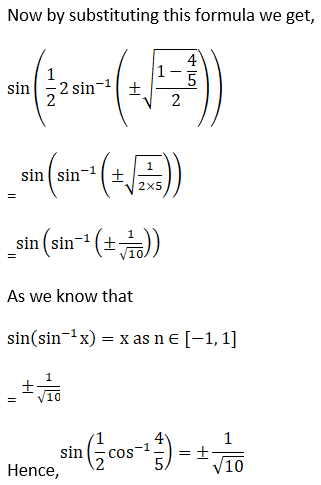(iv) Given Sin (2 tan -1 2/3) + cos (tan-1 √3)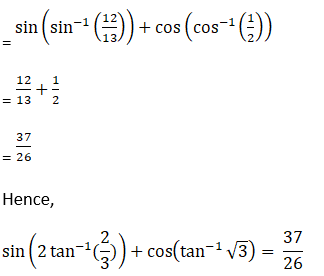2. Prove the following results:

(i) 2 sin-1 (3/5) = tan-1 (24/7)

(ii) tan-1 ¼ + tan-1 (2/9) = ½ cos-1 (3/5) = ½ sin-1 (4/5)

(iii) tan-1 (2/3) = ½ tan-1 (12/5)

(iv) tan-1 (1/7) + 2 tan-1 (1/3) = π/4

(v) sin-1 (4/5) + 2 tan-1 (1/3) = π/2

(vi) 2 sin-1 (3/5) – tan-1 (17/31) = π/4

(vii) 2 tan-1 (1/5) + tan-1 (1/8) = tan-1 (4/7)

(viii) 2 tan-1 (3/4) – tan-1 (17/31) = π/4

(ix) 2 tan-1 (1/2) + tan-1 (1/7) = tan-1 (31/17)

(x) 4 tan-1(1/5) – tan-1(1/239) = π/4

Solution:

(i) Given 2 sin-1 (3/5) = tan-1 (24/7)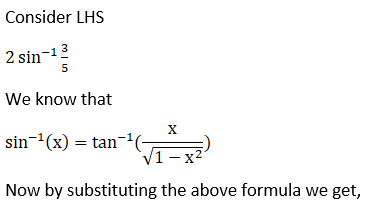Hence, proved.

(ii) Given tan-1 ¼ + tan-1 (2/9) = ½ cos-1 (3/5) = ½ sin-1 (4/5)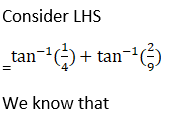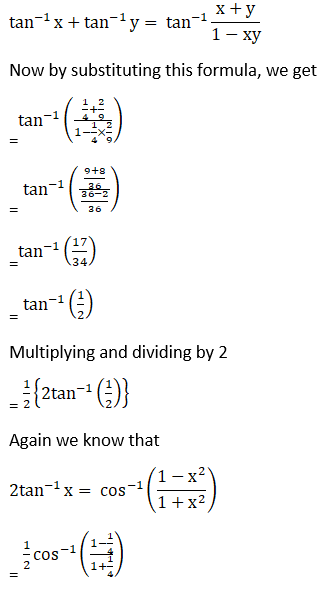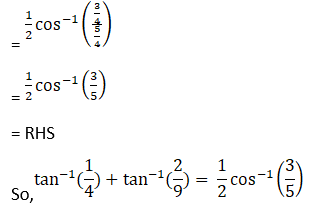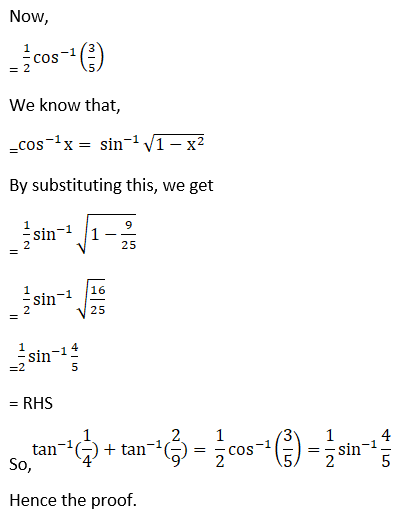Hence, proved.

(iii) Given tan-1 (2/3) = ½ tan-1 (12/5)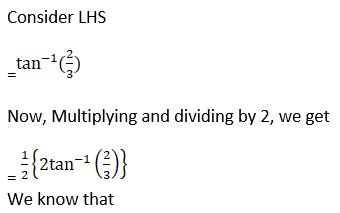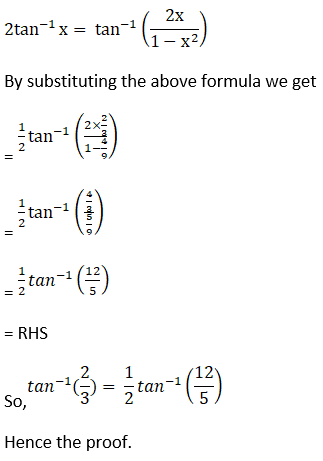Hence, proved.

(iv) Given tan-1 (1/7) + 2 tan-1 (1/3) = π/4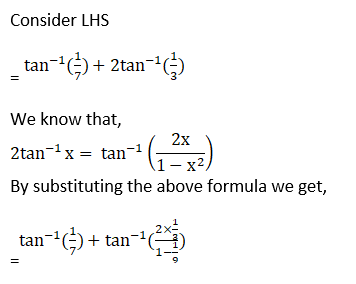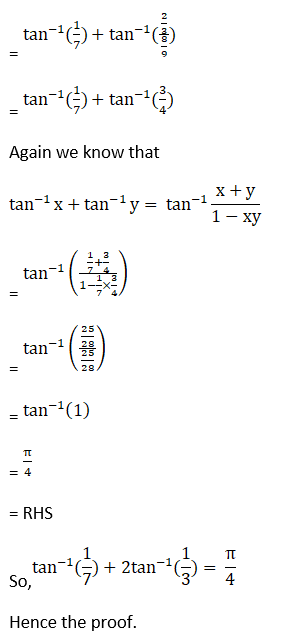Hence, proved.

(v) Given sin-1 (4/5) + 2 tan-1 (1/3) = π/2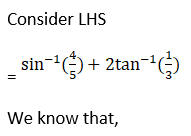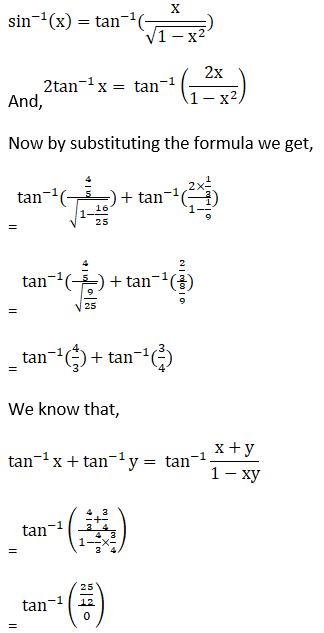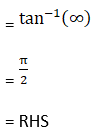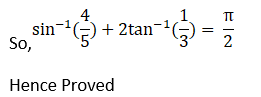(vi) Given 2 sin-1 (3/5) – tan-1 (17/31) = π/4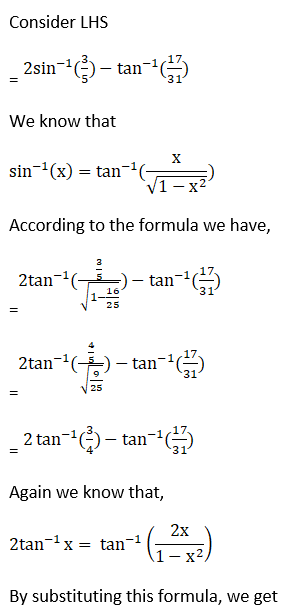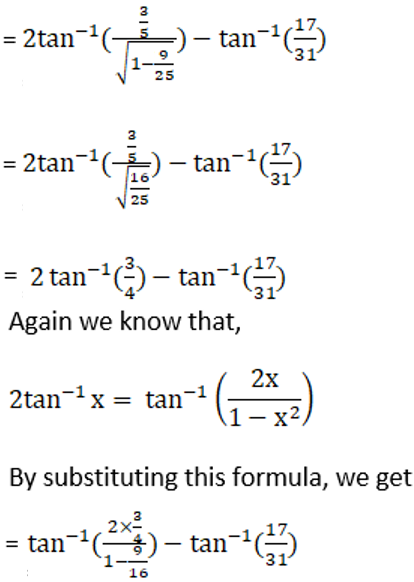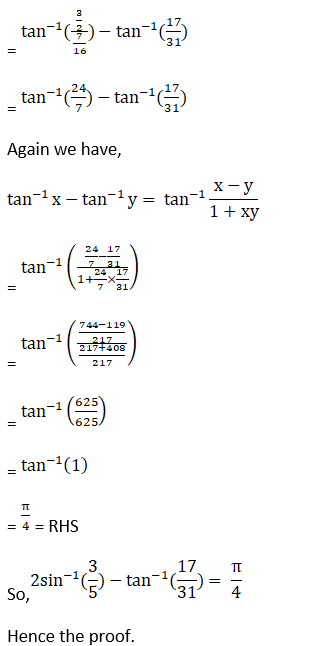(vii) Given 2 tan-1 (1/5) + tan-1 (1/8) = tan-1 (4/7)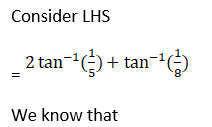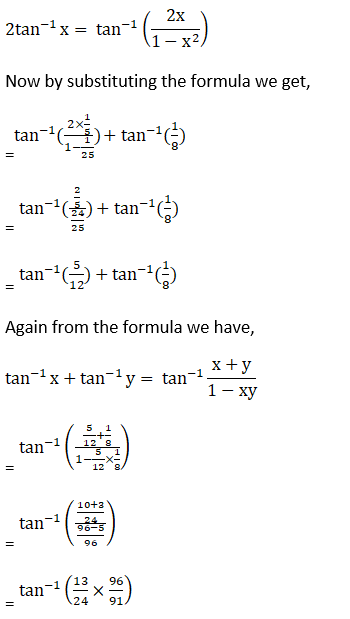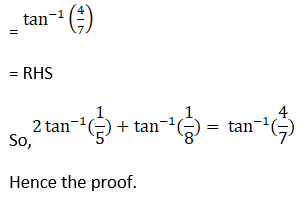Hence, proved.

(viii) Given 2 tan-1 (3/4) – tan-1 (17/31) = π/4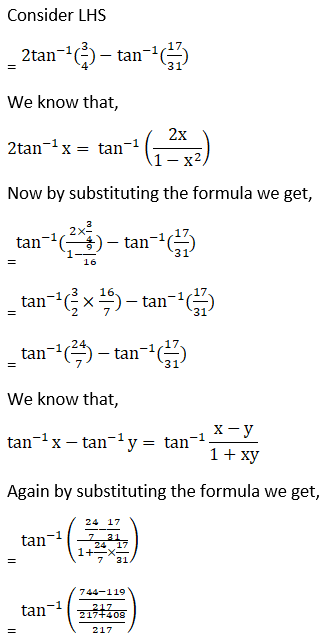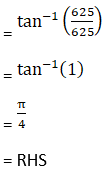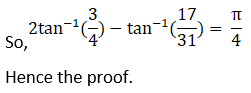Hence, proved.

(ix) Given 2 tan-1 (1/2) + tan-1 (1/7) = tan-1 (31/17)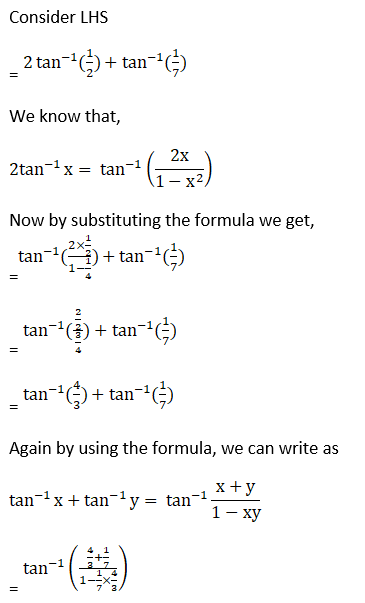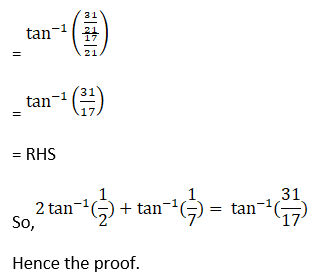Hence, proved.

(x) Given 4 tan-1(1/5) – tan-1(1/239) = π/4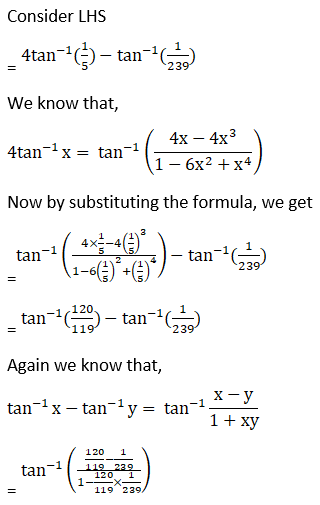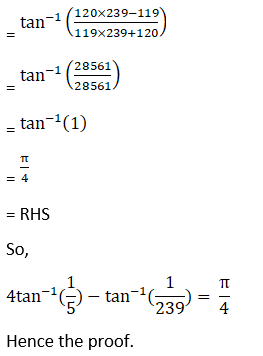Hence, proved.

3. If sin-1 (2a/1 + a2) – cos-1(1 – b2/1 + b2) = tan-1(2x/1 – x2), then prove that x = (a – b)/ (1 + a b)

Solution:

Given sin-1 (2a/1 + a2) – cos-1(1 – b2/1 + b2) = tan-1(2x/1 – x2)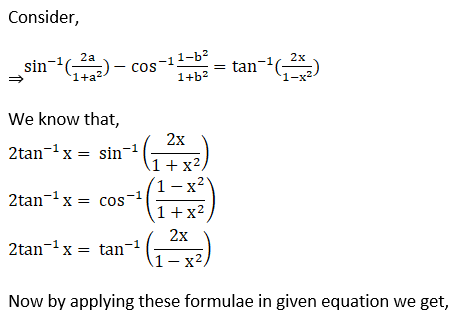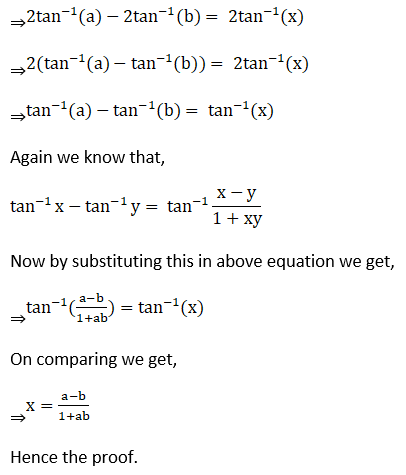Hence, proved.

4. Prove that:

(i) tan-1{(1 – x2)/ 2x)} + cot-1{(1 – x2)/ 2x)} = π/2

(ii) sin {tan-1 (1 – x2)/ 2x) + cos-1 (1 – x2)/ (1 + x2)} = 1

Solution:

(i) Given tan-1{(1 – x2)/ 2x)} + cot-1{(1 – x2)/ 2x)} = π/2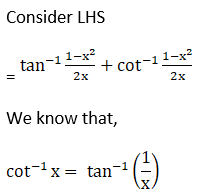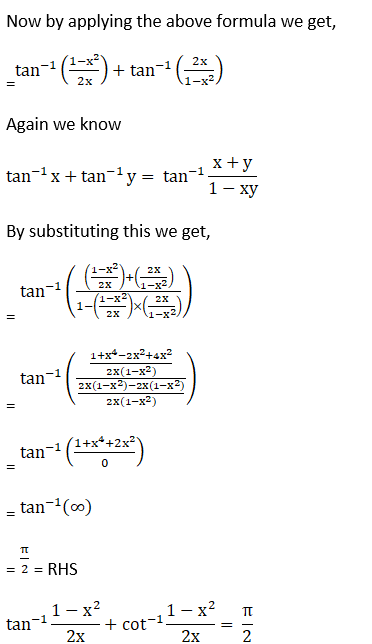Hence, proved.

(ii) Given sin {tan-1 (1 – x2)/ 2x) + cos-1 (1 – x2)/ (1 + x2)}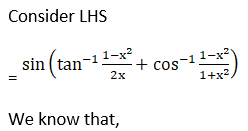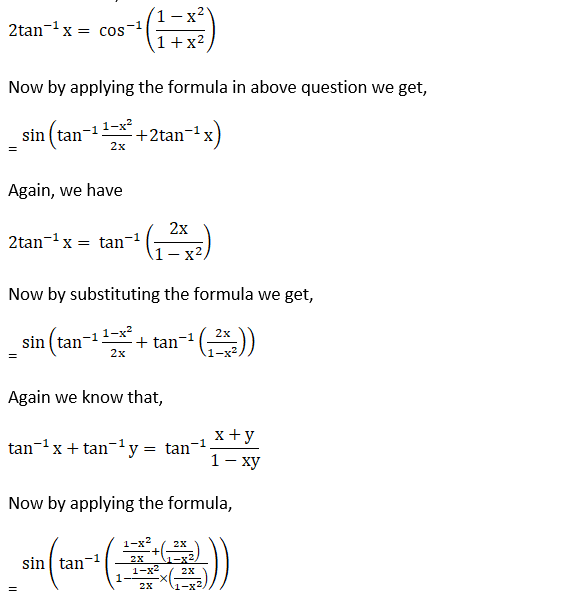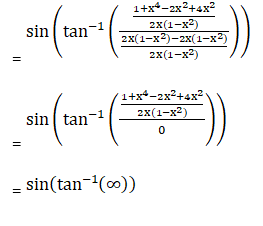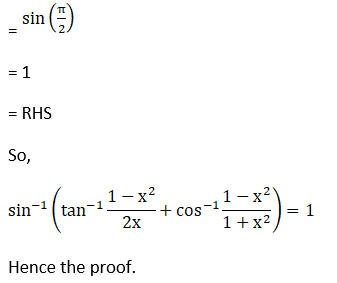Hence, proved.

5. If sin-1 (2a/ 1+ a2) + sin-1 (2b/ 1+ b2) = 2 tan-1 x, prove that x = (a + b/ 1 – a b)

Solution:

Given sin-1 (2a/ 1+ a2) + sin-1 (2b/ 1+ b2) = 2 tan-1 x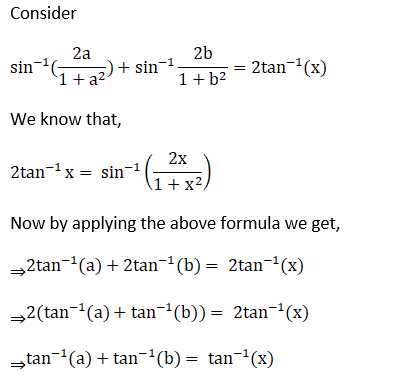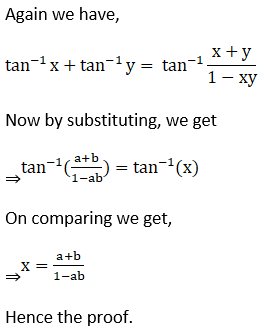Hence, proved.

### Access other exercises of RD Sharma Solutions For Class 12 Chapter 4 – Inverse Trigonometric Functions

Exercise 4.1 Solutions

Exercise 4.2 Solutions

Exercise 4.3 Solutions

Exercise 4.4 Solutions

Exercise 4.5 Solutions

Exercise 4.6 Solutions

Exercise 4.7 Solutions

Exercise 4.8 Solutions

Exercise 4.9 Solutions

Exercise 4.10 Solutions

Exercise 4.11 Solutions

Exercise 4.12 Solutions

Exercise 4.13 Solutions